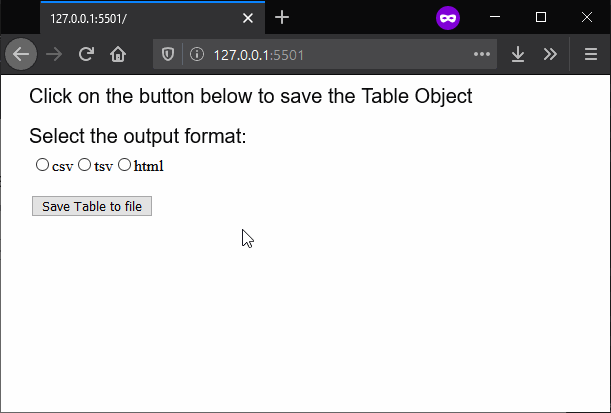# p5.js | saveTable() Function

• Last Updated : 16 Mar, 2020

The saveTable() function is used to save a p5.Table object to a file. The format of the file saved can be defined as a parameter to the function. By default, it saves a text file with comma-separated-values, however, it can be used to save it using-tab separated-values or generate an HTML table from it.

Syntax:

`saveTable( Table, filename, options )`

Parameters: This function accept three parameters as mentioned above and described below:

• Table: This is a p5.Table object that would be saved to the file.
• filename: It specifies the string that is used as the filename of the saved file.
• options: It is a string which denotes the format of the table to be saved. It can be either “csv” which saves the table using comma-separated-values, “tsv” which saves the table using tab-separated-values, or “html” which generates an HTML table. It is an optional parameter.

Below example illustrates the saveTable() function in p5.js:

Example:

 `function` `setup() {``  ``createCanvas(600, 300);``  ``textSize(20);``  ` `  ``text(``"Click on the button below to "``        ``+ ``"save the Table Object"``, 20, 20);``   ` `  ``text(``"Select the output format:"``, 20, 60);``  ` `  ``// Create radio button for choosing``  ``// file format to save the table``  ``radio = createRadio();``  ``radio.position(30, 80);``  ``radio.option(``'csv'``);``  ``radio.option(``'tsv'``);``  ``radio.option(``'html'``);``  ` `  ``// Create a button for saving the Table object``  ``saveBtn = createButton(``"Save Table to file"``);``  ``saveBtn.position(30, 120);``  ``saveBtn.mousePressed(saveFile);``  ` `  ``// Create the table for saving to file``  ``table = ``new` `p5.Table();``  ` `  ``table.addColumn(``'Invention'``);``  ``table.addColumn(``'Inventors'``);``  ` `  ``let tableRow = table.addRow();``  ``tableRow.setString(``'Invention'``, ``'Telescope'``);``  ``tableRow.setString(``'Inventors'``, ``'Galileo'``);``  ` `  ``tableRow = table.addRow();``  ``tableRow.setString(``'Invention'``, ``'Steam Engine'``);``  ``tableRow.setString(``'Inventors'``, ``'James Watt'``);``  ` `  ``tableRow = table.addRow();``  ``tableRow.setString(``'Invention'``, ``'Radio'``);``  ``tableRow.setString(``'Inventors'``, ``'Guglielmo Marconi'``);``}``  ` `function` `saveFile() {``  ` `  ``// Get the output format selected``  ``// from the radio buttons``  ``outputFormat = radio.value();``  ` `  ``// Save the table to file with the given format``  ``saveTable(table, ``'tableOutput'``, outputFormat);``}`

Output:Online editor: https://editor.p5js.org/

My Personal Notes arrow_drop_up